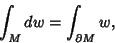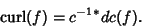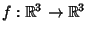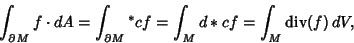## Stokes' Theorem

Fora Differential k-Form with compact support on an oriented-dimensional Manifold,(1)

whereis the Exterior Derivative of the differential form. This connects to the standard'' Gradient, Curl, and Divergence Theorems by the following relations. Ifis a function on,(2)

where(the dual space) is the duality isomorphism between a Vector Space and its dual, given by the Euclidean Inner Product on. Ifis a Vector Field on a,(3)

whereis the Hodge Star operator. Ifis a Vector Field on,(4)

With these three identities in mind, the above Stokes' theorem in the three instances is transformed into the Gradient, Curl, and Divergence Theorems respectively as follows. Ifis a function onandis a curve in, then(5)

which is the Gradient Theorem. Ifis a Vector Field andan embedded compact 3-manifold with boundary in, then(6)

which is the Divergence Theorem. Ifis a Vector Field andis an oriented, embedded, compact 2-Manifold with boundary in, then(7)

which is the Curl Theorem.

Physicists generally refer to the Curl Theorem(8)

as Stokes' theorem.